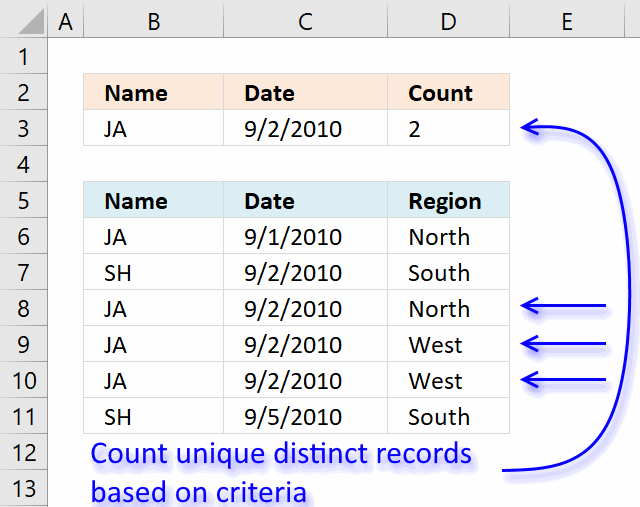Author: Oscar Cronquist Article last updated on January 19, 2019re: Count records between two dates and a criterion

based on the example, i was looking for 1 date and 1 criterion. i slightly modify the formula to

=SUMPRODUCT(--(\$B\$1:\$B\$9=\$E\$2), --(\$A\$1:\$A\$9=\$E\$3)) + ENTER

[assuming E2 = 9-2-2010]

the result would be 1 (one 'JA' found on 9-2-2010 date)

but this is summation of records found on 1 date with 1 criterion. It will not work if there is *multiple* 'JA' criterion exist on the same date because SUMPRODUCT summed up the records found.

I'm curious to know...

1) What if I want to know the UNIQUE DISTINCT records found on 1 date with 1 criterion?

2) Working on >100k rows of data, this formula literally slows down Excel (heavy calculation and recalculations). Is there an alternative to speed it up? UDF? array formula?

thanks!

Array formula in cell D3:

=SUM(IF((\$B\$6:\$B\$11=B3)*(\$C\$6:\$C\$11=C3), (1/COUNTIFS(\$B\$6:\$B\$11, \$B\$6:\$B\$11, \$C\$6:\$C\$11, \$C\$6:\$C\$11, \$D\$6:\$D\$11, \$D\$6:\$D\$11)), 0))

### How to create an array formula

1. Copy (Ctrl + c) and paste (Ctrl + v) array formula into formula bar.
2. Press and hold Ctrl + Shift.
3. Press Enter once.
4. Release all keys.

### How the array formula in cell D3 works

Step 1 - Count records

The COUNTIFS function calculates the number of cells across multiple ranges that equals all given conditions.

COUNTIFS(\$B\$6:\$B\$11, \$B\$6:\$B\$11, \$C\$6:\$C\$11, \$C\$6:\$C\$11, \$D\$6:\$D\$11, \$D\$6:\$D\$11)

becomes

COUNTIFS({"JA";"SH";"JA"; "JA";"JA";"SH"}, {"JA";"SH";"JA"; "JA";"JA";"SH"}, {40422;40423; 40423;40423;40423;40426}, {40422;40423; 40423;40423;40423;40426}, {"North";"South";"North"; "West";"West";"South"}, {"North";"South";"North"; "West";"West";"South"})

and returns array {1;1;1;2;2;1}

Step 2 - Filter records using name and date criteria

The IF function returns one value (argument2) if TRUE and another (argument3) if FALSE.

IF((\$B\$6:\$B\$11=B3)*(\$C\$6:\$C\$11=C3), (1/COUNTIFS(\$B\$6:\$B\$11, \$B\$6:\$B\$11, \$C\$6:\$C\$11, \$C\$6:\$C\$11, \$D\$6:\$D\$11, \$D\$6:\$D\$11)), 0)

becomes

IF((\$B\$6:\$B\$11=B3)*(\$C\$6:\$C\$11=C3), (1/{1;1;1;2;2;1}), 0)

becomes

IF(({"JA";"SH";"JA";"JA";"JA";"SH"}="JA")*({40422;40423;40423;40423;40423;40426}=40423), (1/{1;1;1;2;2;1}), 0)

becomes

IF(({0;0;1;1;1;0}, (1/{1;1;1;2;2;1}), 0)

becomes

IF(({0;0;1;1;1;0}, {1;1;1;0,5;0,5;1}, 0)

and returns {0;0;1;0,5;0,5;0}

Step 3 - Sum values

The SUM function adds numbers an return the total.

=SUM(IF((\$B\$6:\$B\$11=B3)*(\$C\$6:\$C\$11=C3), (1/COUNTIFS(\$B\$6:\$B\$11, \$B\$6:\$B\$11, \$C\$6:\$C\$11, \$C\$6:\$C\$11, \$D\$6:\$D\$11, \$D\$6:\$D\$11)), 0))

becomes

=SUM({0;0;1;0,5;0,5;0}) and returns 2 in cell D3.